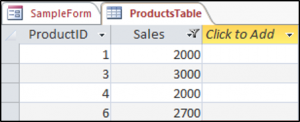# VBA Tables and ListObjects

This tutorial will demonstrate how to work with Tables and ListObjects in VBA.

## VBA Tables and ListObjects

Tables are one of Excel’s most useful and powerful features, in this tutorial, we will go over how to use VBA to create a table, add a simple sort to a table, filter a table and perform other table-related tasks.

### Create a Table With VBA

The ListObjects.Add Method can add a table to a worksheet, based on a range in that worksheet. We have the range shown in (\$A\$1:\$B\$8) on a worksheet called Sheet1.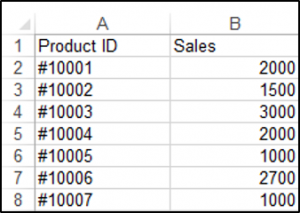The following code will add a table, called Table1 to your worksheet, based on the range (\$A\$1:\$B\$8) using the default Table Style:

``````Sub CreateTableInExcel()

ActiveWorkbook.Sheets("Sheet1").ListObjects.Add(xlSrcRange, Range("\$A\$1:\$B\$8"), , xlYes).Name = _
"Table1"

End Sub``````

The result is: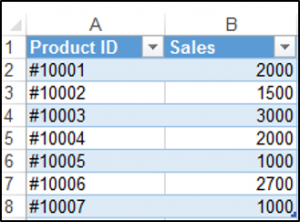### Inserting a Column at the End of the Table with VBA

You can use the ListColumns.Add method in order to add a column to the end of your table. We have our table called Table1 shown below.You can add a column to your table using the following code, which will always add a column to the end of the table:

``````Sub AddColumnToTheEndOfTheTable()

End Sub``````

The result is: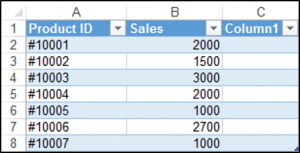### Inserting a Row at the Bottom of the Table with VBA

You can use the ListRows.Add method to add a row to the bottom of your table. We have our table called Table1 shown below.The following code will always add a row to the bottom of your table.

``````Sub AddRowToTheBottomOfTheTable()

End Sub``````

The result is: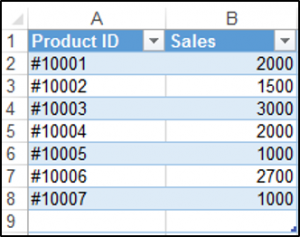### Adding a Simple Sort with VBA

You can sort a table with VBA. We have our table called Table1 shown below and we can use VBA to sort the Sales Column from lowest to highest.The following code will sort the Sales column in ascending order.

``````Sub SimpleSortOnTheTable()

ActiveWorkbook.Worksheets("Sheet1").ListObjects("Table1").Sort.SortFields.Clear
Key:=Range("Table1[[#All],[Sales]]"), SortOn:=xlSortOnValues, Order:= _
xlAscending, DataOption:=xlSortNormal

With ActiveWorkbook.Worksheets("Sheet1").ListObjects("Table1").Sort

.MatchCase = False
.Orientation = xlTopToBottom
.SortMethod = xlPinYin
.Apply

End With

End Sub``````

The result is: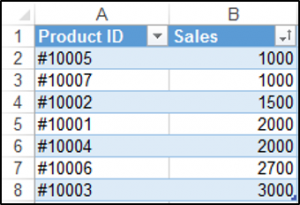### Filter a Table With VBA

You can also filter an Excel table using VBA. We have our table called Table1 and we would like to filter the table so that only sales of greater than 1500 are shown.We can use the Autofilter method, which has five optional parameters. Since we’d like to filter the Sales column which is the second column we set the Field to 2, and we use the xlAnd operator parameter, which is used for dates and numbers.

``````Sub SimpleFilter()

ActiveWorkbook.Sheets("Sheet1").ListObjects("Table1").Range.AutoFilter Field:=2, Criteria1:= _
">1500", Operator:=xlAnd

End Sub``````

The result is: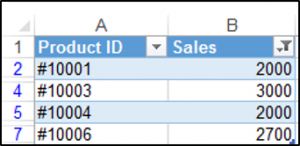Stop searching for VBA code online. Learn more about AutoMacro - A VBA Code Builder that allows beginners to code procedures from scratch with minimal coding knowledge and with many time-saving features for all users!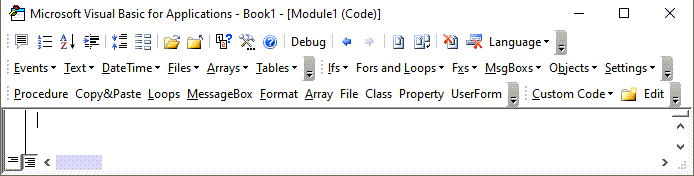### Clear the Filter with the ShowAllData Method in VBA

You can access the ShowAllData Method of the Worksheet class in order to clear the filter. If it’s a table’s filter(s) that you want to clear, then you first have to select a cell in the table, which you can do in VBA.

The ShowAllData method will generate an error if one does not use conditional logic in order to check if there has been a filter applied in the worksheet. The following code shows you how to do this:

``````Sub ClearingTheFilter()

If ActiveWorkbook.Worksheets("Sheet1").FilterMode = True Then
ActiveSheet.ShowAllData

End If

End Sub``````

### Clear All Filters From An Excel Table

You can access the ShowAllData Method of the ListObject class without having to select a cell in the table first. The following code shows you how to do this:

``````Sub ClearAllTableFilters()
ActiveWorkbook.Worksheets("Sheet1").ListObjects("Table1").AutoFilter.ShowAllData
End Sub``````

### Deleting A Row With VBA

You can delete a row in the databody of your table using the ListRows.Delete method. You have to specify which row using the row number. We have the following table called Table1.Let’s say you wanted to delete the second row in the databody of your table, the following code would allow you to do this:

``````Sub DeleteARow()

ActiveWorkbook.Worksheets("Sheet1").ListObjects("Table1").ListRows(2).Delete

End Sub``````

The result is: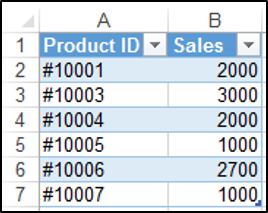VBA Programming | Code Generator does work for you!

### Deleting a Column With VBA

You can delete a column from your table using the ListColumns.Delete method. We have the following table called Table1 shown below:In order to delete the first column, you would use the following code:

``````Sub DeleteAColumn()

ActiveWorkbook.Worksheets("Sheet1").ListObjects("Table1").ListColumns(1).Delete

End Sub``````

The result is: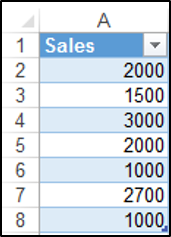### Converting a Table Back to a Range in VBA

You can convert a table back to a normal range using VBA. The following code shows you how to convert a table called Table1 back to a range:

``````Sub ConvertingATableBackToANormalRange()

ActiveWorkbook.Sheets("Sheet1").ListObjects("Table1").Unlist

End Sub``````

### Adding Banded Columns and formatting to all the Tables in a Worksheet using VBA

You can access all the tables in your worksheet using the ListObjects collection. In the sheet below we have two tables and we would like to add a Banded Column to both the tables at once and change the font of the data section of both tables to bold, using VBA.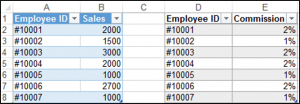``````Sub AddingBandedColumns()

Dim tbl As ListObject
Dim sht As Worksheet

Set sht = ThisWorkbook.ActiveSheet

For Each tbl In sht.ListObjects
tbl.ShowTableStyleColumnStripes = True
tbl.DataBodyRange.Font.Bold = True
Next tbl

End Sub``````

The result is: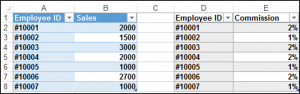### Creating a Table in Access in VBA Using DoCmd.RunSQL

One of the main ways to create a table in Access in VBA, is through using the DoCmd.RunSQL method to run an action query with a SQL statement.

We have a button on our sample form and when we click on the button we’d like to create a table called ProductsTable with two fields or columns, one would be the primary key field called ProductsID and the other would be a field called Sales.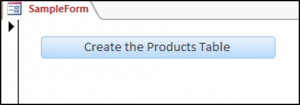In order to create this table we would use the following code:

``````Private Sub cmdCreateProductsTable_Click()

DoCmd.RunSQL "CREATE TABLE ProductsTable " _
& "(ProductID INTEGER PRIMARY KEY, Sales Integer);"

End Sub``````

The result is: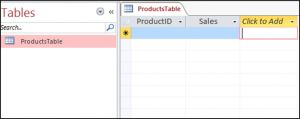### Filtering a Table in Access Using VBA

You can also filter a table in Access using the DoCmd.ApplyFilter method. We have our simple table shown below in Access called ProductsTable.

We would like to press this button on our form and then only see Sales that are greater than 1500.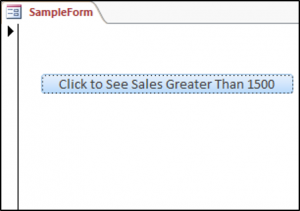So, we would use the following code to do this:

``````Private Sub cmdFilter_Click()

DoCmd.OpenTable "ProductsTable"

DoCmd.ApplyFilter , "[Sales]>1500"

End Sub``````

The result is: